##### ASVAB AFQT For Dummies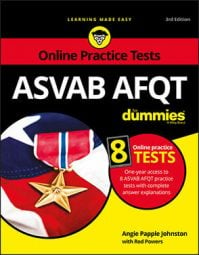If you're thinking about joining the U.S. military, your Armed Forces Qualification Test (AFQT) score may well be the most important score you achieve on any military test. You need a qualifying score on the AFQT, or your plans for enlistment come to a dead end — and each branch of the military has its own minimum AFQT score requirements.

Part of getting a high score on the AFQT involves brushing up on your math skills. You need to memorize key formulas and use proven test-taking strategies to maximize your chances for a high math score. The other part is making sure you have a firm grasp on English; in order to ace the language parts of the AFQT, you need a solid vocabulary and good reading comprehension skills.

## ASVAB AFQT qualifying scores

To enlist in the U.S. military, you have to take the Armed Services Vocational Aptitude Battery (ASVAB) exam, which consists of nine separately timed subtests. These subtests are used by the military to determine your aptitude to learn various military jobs.

Four of the ASVAB subtests are used to compute the Armed Forces AFQT score, a percentile score that runs from 1 to 99. This score determines whether you’re qualified to join the military service of your choice. Each branch of the military has its own minimum AFQT score standards:

• Army (including Army National Guard and Army Reserves): The Army requires a minimum AFQT score of 31 for those with a high school diploma and a score of 50 for those with a high school equivalency certificate. When the Army is experiencing high recruiting and reenlistment rates, it has been known to temporarily increase its qualifying AFQT score minimum to as high as 50.
• Air Force (including Air National Guard and Air Force Reserves): Air Force recruits must score at least a 36 on the AFQT to qualify for enlistment, but more than 70 percent of people who are accepted for an Air Force enlistment score 50 or above. For those who have a high school equivalency certificate instead of a high school diploma, the minimum score is 65.
• Navy: Navy recruits must score at least 35 on the AFQT to qualify for enlistment. If you have a high school equivalency certificate, the minimum score is 50.
• Navy Reserves: The Navy Reserves requires a minimum score of 31 on the AFQT for those with a high school diploma and 50 for those with a high school equivalency certificate. The Navy is the only branch for which the requirements for the Reserves are different from the requirements for the branch itself.
• Marine Corps (including Marine Corps Reserves): Marine Corps recruits must score at least 32. People who have a high school equivalency certificate must score a minimum of 50 on the AFQT to be considered. The Marine Corps limits GED enlistments to about 5 percent to 10 percent per year.
• Coast Guard (including Coast Guard Reserves): The Coast Guard requires a minimum of 40 points on the AFQT. A waiver is possible for applicants with prior service if their ASVAB line scores qualify them for a specific job and they’re willing to enlist in that job. The minimum AFQT score for people who have a high school equivalency certificate is 50.

## Acing the math subtests

Two of the four subtests that make up your AFQT score are math tests. The first, Arithmetic Reasoning, tests your ability to use mathematics to solve various problems that you may encounter in real life — in other words, math word problems. The second, Mathematics Knowledge, tests your ability to solve general math problems. Here are some tips to help maximize your ASVAB math subtest scores:

• Memorize the math order of operations. Start by working everything in parentheses. Then move on to exponents. Then perform any multiplication and division. End with addition and subtraction. An easy way to remember this order is to think of the phrase, “Please Excuse My Dear Aunt Sally” (Parentheses, Exponents, Multiply, Divide, Add, Subtract). While this order is flexible in some situations, knowing the basic order will help you maximize your score.

• Remember the apples-and-oranges rule. Be sure to convert units of measurement so that they’re consistent. If a question asks how many 3-x-6-inch bricks it will take to cover a 6-x-6-foot patio, be sure to recognize that inches and feet are two different measurements and perform the necessary conversions.

• Memorize common math formulas before the day of the test. These formulas are not provided for you at the testing center. After you receive your scratch paper, take a moment before the test starts and write down all the formulas you’ve memorized. Here’s a list of some of the formulas you need to know:

• Perimeter of a square: p = 4s, where s = one side of the square

• Area of a square: a = s2

• Diagonal of a square: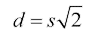• Perimeter of a rectangle: p = 2l + 2w, where l = the length and w = the width of the rectangle

• Area of a rectangle: a = lw

• Diagonal of a rectangle: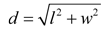, where d = the diagonal, l = the length, and w = the width of the rectangle. This formula is the Pythagorean theorem solved for the hypotenuse (c) — it just uses different letters.

• Perimeter of a triangle: p = s1 + s2 + s3, where s = the length of each leg of the triangle

• Area of a triangle: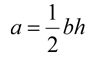, where b = the length of the triangle’s base (bottom) and h = the height of the triangle

• Pythagorean theorem: a2 + b2 = c2, where c equals the length of a right triangle’s hypotenuse, and a and b equal the lengths of the remaining two legs of the right triangle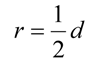where d = the diameter of the circle

• Diameter of a circle: d = 2r

• Circumference of a circle: c = 2Πr

• Area of a circle: a = Πr2

• Volume of a cube: v = s3, where s = the length of one side of the cube

• Volume of a rectangular box: v = lwh, where l = the length, w = the width, and h = the height of the box

• Volume of a cylinder: v = Πr2h, where r = the radius of the cylinder and h = the height of the cylinder

• Surface area of a cube: SA = 6s2

• Surface area of a rectangular box: SA =2lw + 2wh + 2lh

• Use the scratch paper provided to help you solve problems. Draw a picture for math word problems to help you visualize the situation and pick out the relevant information. Remember: You can’t use a calculator on any of the AFQT subtests.

• For difficult problems, try plugging the possible answer choices into the equation to see which one is right.

• Learn to separate the meat from the fat, and select only the information needed to solve the problem. Math word problems often provide extra information to confuse you.